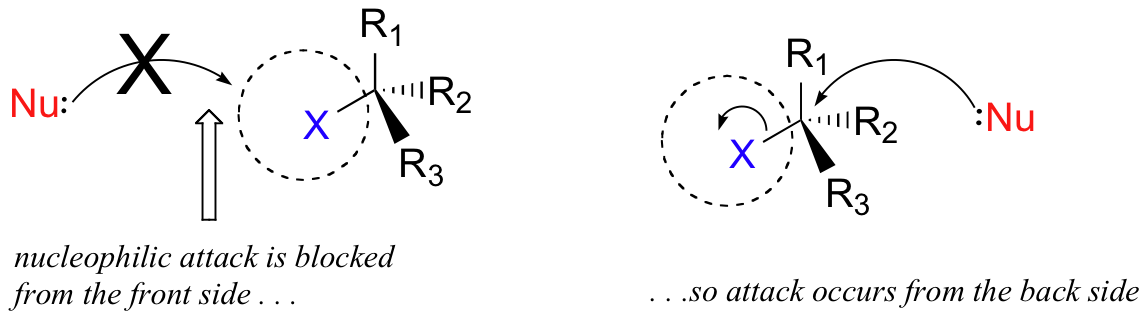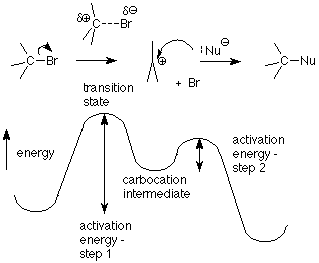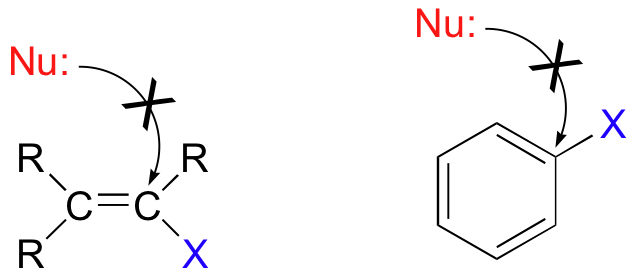# 8.2: Physical chemistry for SN2 and SN1 reactions

$$\newcommand{\vecs}{\overset { \rightharpoonup} {\mathbf{#1}} }$$ $$\newcommand{\vecd}{\overset{-\!-\!\rightharpoonup}{\vphantom{a}\smash {#1}}}$$$$\newcommand{\id}{\mathrm{id}}$$ $$\newcommand{\Span}{\mathrm{span}}$$ $$\newcommand{\kernel}{\mathrm{null}\,}$$ $$\newcommand{\range}{\mathrm{range}\,}$$ $$\newcommand{\RealPart}{\mathrm{Re}}$$ $$\newcommand{\ImaginaryPart}{\mathrm{Im}}$$ $$\newcommand{\Argument}{\mathrm{Arg}}$$ $$\newcommand{\norm}{\| #1 \|}$$ $$\newcommand{\inner}{\langle #1, #2 \rangle}$$ $$\newcommand{\Span}{\mathrm{span}}$$ $$\newcommand{\id}{\mathrm{id}}$$ $$\newcommand{\Span}{\mathrm{span}}$$ $$\newcommand{\kernel}{\mathrm{null}\,}$$ $$\newcommand{\range}{\mathrm{range}\,}$$ $$\newcommand{\RealPart}{\mathrm{Re}}$$ $$\newcommand{\ImaginaryPart}{\mathrm{Im}}$$ $$\newcommand{\Argument}{\mathrm{Arg}}$$ $$\newcommand{\norm}{\| #1 \|}$$ $$\newcommand{\inner}{\langle #1, #2 \rangle}$$ $$\newcommand{\Span}{\mathrm{span}}$$$$\newcommand{\AA}{\unicode[.8,0]{x212B}}$$

# The SN2 reaction

There are two mechanistic models for how a nucleophilic substitution reaction can proceed at an alkyl halide (or similar) – SN2 and SN1. In the first picture, SN2, the reaction takes place in a single step, and bond-forming and bond-breaking occur simultaneously.This is called an ‘associative’, or ‘SN2′ mechanism.  In the term SN2,  S stands for ‘substitution’, the subscript N stands for ‘nucleophilic’, and the number  2 refers to the fact that this is a bimolecularreaction: the overall rate depends on a step in which two separate molecules (the nucleophile and the electrophile) collide. A potential energy diagram for this reaction shows the transition state (TS) as the highest point on the pathway from reactants to products.If you look carefully at the progress of the SN2 reaction, you will realize something very important about the outcome. The nucleophile, being an electron-rich species, must attack the electrophilic carbon from the back side relative to the location of the leaving group.  Approach from the front side simply doesn’t work: the leaving group  – which is also an electron-rich group – blocks the way.The result of this backside attack is that the stereochemical configuration at the central carbon inverts as the reaction proceeds.  In a sense, the molecule is turned inside out. At the transition state, the electrophilic carbon and the three ‘R’ substituents all lie on the same plane.What this means is that SN2 reactions whether enzyme catalyzed or not, are inherently stereoselective: when the substitution takes place at a stereocenter, we can confidently predict the stereochemical configuration of the product.

### Exercise

Predict the structure of the product in this SN2 reaction.  Be sure to specify stereochemistry.[hidden-answer a=”659030″]Notice that the reaction occurs with inversion of configuration: the leaving group (I) was pointing out of the plane of the page, while the nucleophile (CH3S) attacks from behind, and ends up pointing into the plane of the page.### Rate law for the SN2 reaction

Earlier we saw that the energy required to reach the transition state comes from the energy with which the nucleophile and the alkyl halide collide. The requirement for a collision also means that the frequency with which the nucleophile and the alkyl halide collide is important. This frequency is primarily controlled by concentration.If the concentration of the alkyl halide is high, then there will be many opportunities for a nucleophile to collide with an alkyl halide molecule. The rate of the reaction will increase proportionately as the alkyl halide concentration is made higher. When this is the case the reaction is said to be first order in alkyl halide. Similarly an increase in the nucleophile concentration will result in a proportionate increase in the rate, so the reaction is also first order in nucleophile. Overall, the rate depends on the concentration of both reactants, and the reaction is said to be second order. This can be summarized in the rate equation or rate law.

Rate = k[RX][Nu]

It is the second order behavior (requirement for two molecules to collide in the critical transition state) which is designated by the “2” in SN2.

### The SN2 reaction

In summary, we see that for the SN2 reaction:

• The reaction is “concerted” – it has only one step in the mechanism
• The reaction rate depends on the concentration of both the electrophile (the alkyl halide or similar) and the nucleophile.
• The nucleophile attacks the “back side” of the electrophile, with the leaving group leaves from the “front”.  This also means that if the leaving group is leaving from a chiral carbon, the stereochemistry at that center is inverted,

# The SN1 reaction

A second model for a nucleophilic substitution reaction is called the ‘dissociative’, or ‘SN1′ mechanism: in this picture, the C-X bond breaks first, before the nucleophile approaches:This results in the formation of a carbocation: because the central carbon has only three bonds, it bears a formal charge of +1. Recall that a carbocation should be pictured as sp2hybridized, with flat (trigonal planar) geometry.  Perpendicular to the plane formed by the three sp2 hybrid orbitals is an empty, unhybridized p orbital.In the second step of this two-step reaction, the nucleophile attacks the empty, ‘electron hungry’ p orbital of the carbocation to form a new bond and return the carbon to tetrahedral geometry.We saw that SN2 reactions result specifically in inversion of stereochemistry at the electrophilic carbon center.  What about the stereochemical outcome of SN1 reactions? In the model SN1 reaction shown above, the leaving group dissociates completely from the vicinity of the reaction before the nucleophile begins its attack.  Because the leaving group is no longer in the picture, the nucleophile is free to attack from either side of the planar, sp2-hybridized carbocation electrophile.  This means that about half the time the product has the same stereochemical configuration as the starting material (retention of configuration), and about half the time the stereochemistry has been inverted.  In other words, the product has become racemic and optically inactive. As an example, the tertiary alkyl bromide below would be expected to form a racemic mixture of R and S alcohols after an SN1 reaction with water as the incoming nucleophile.### Exercise

Draw the structure of the intermediate in the two-step nucleophilic substitution reaction above.

The intermediate species is a carbocation that forms after the bromine leaves:In the SN1 reaction we see an example of a reaction intermediate, a very important concept in the study of organic reaction mechanisms that was first introduced in Chapter 5  Recall that many important organic reactions do not occur in a single step; rather, they are the sum of two or more discrete bond-forming / bond-breaking steps, and involve transient intermediate species that go on to react very quickly. The SN1 reaction involves both a heterolysis step and a coordination step, as well as (usually) at least one acid-base step.  The carbocation species is the reactive intermediate in the SN1 reaction. A potential energy diagram for an SN1 reaction shows that the carbocation intermediate can be visualized as a kind of “mountain valley” in the path of the reaction, higher in energy than both the reactant and product but lower in energy than the two transition states.### Exercise

Draw structures representing  TS1 and TS2 in the reaction above.  Use the solid/dash wedge convention to show three dimensions.

[hidden-answer a=”607961″][/hidden-answer]

Recall (from Hammond’s postulate) that the first heterolysis step of the reaction above, in which two charged species are formed from a neutral molecule, is much the slower of the two steps, and is therefore rate-determining.  This is illustrated by the energy diagram, where the activation energy for the first step is higher than that for the second step. Also recall that an SN1 reaction has first order kinetics, because the rate determining step involves one molecule splitting apart, not two molecules colliding.

### Exercise

Consider two nucleophilic substitutions that occur uncatalyzed in solution.  Assume that reaction A is SN2, and reaction B is SN1.  Predict, in each case, what would happen to the rate of the reaction if the concentration of the nucleophile were doubled, while all other conditions remained constant.Reaction A is a concerted (one-step) reaction, essentially a collision between two species.  Therefore, if the concentration of nucleophile (CH3S) were doubled in the solution, the rate of the reaction should also double. You should recall learning about rate expressions in your general chemistry course – this is an example of a second order rate expression, where the rate depends on some rate constant (k) and the product of two concentration:

rate = k[CH3I] [CH3S]

(If this is unfamiliar to you,  now would be a good time for a quick review of rate expressions in your general chemistry textbook!)

Reaction B is a two-step reaction.  The first step – the breaking of the C-Br bond – is the slow, rate determining step, and does not involve the CH3SH nucleophile at all.  Therefore, changing the concentration of the nucleophile should have no effect on the rate of the reaction. (If the concentration of CH3SH were doubled, the second step would occur twice as fast – but the rate of the first, slower step would not change, and so the overall rate of the reaction would not be affected).  The rate expression in this case is first order (it depends on the concentration of only one reactant):

rate = k[C(CH3)3Br]

Many SN1 reactions are of a class that are referred to as solvolysis, where a solvent molecule participates in the reaction as a nucleophile. The SN1 reaction of allyl bromide in methanol is an example of what we would call methanolysis, while if water is the solvent the reaction would be called hydrolysis.  In reactions like this that use an uncharged nucleophile, an acid-base step is needed after the coordination step, in order to remove H+ and form the uncharged final product.Because water and alcohols are relatively weak nucleophiles, they are less likely to react in an SN2 fashion.

### Rate law for the SN1 reaction

The “1” in SN1 means that only one molecule needs to be “activated” in order to reach the transition state. That molecule is the alkyl halide. The critical step in this mechanism is the first heterolysis step, in which the bond between the carbon atom and the halogen leaving group is broken. The transition state for this step has the bond stretched far enough that the halide ion is balanced between leaving as a stable chloride or bromide ion or slipping back into a covalent bond.(The energy required to break this bond comes from random collisions with the solvent without the solvent reacting.) The activation energy for the first step is higher than for the second step (coordination), so the rate of the reaction is controlled by how many molecules get through the first step. Once a molecule is through the first step, it can react rapidly in the second step. We call the step which is slowest the “rate determining step.” Notice that the rate determining step for this reaction doesn’t involve the nucleophile. That means that changing the concentration of the nucleophile doesn’t affect the rate. The only concentration which affects the rate is the concentration of the alkyl halide. We thus have a first order reaction, and the rate law includes only one reactant:

Rate = k[RX]

This also means that the strength of the nucleophile — its ability to use its electron pair to make a bond — isn’t important in determining how fast the reaction goes. It’s not involved in the rate determining step, so it has no effect on the energy of that transition state.

### The SN1 reaction

In summary, for the SN1 reaction

• The reaction involves at least two steps – heterolysis (to form the carbocation) and coordination (when the nucleophile bonds to the carbon).  In addition, there are often acid-base steps needed in order to give uncharged products at the end.
• Because the rate determining step is the heterolysis step, the rate depends only on the alkyl halide, and the nucleophile has no effect on rate.  This means that weak nucleophiles can work well in the SN1 reaction.
• The nucleophile is free to approach the flat carbocation equally from either side in the coordination step.  This means that if the substitution is occurring at a (single) chiral carbon, the product will be racemic (a 50:50 mixture of enantiomers).

### Exercise

Draw a complete curved-arrow mechanism for the methanolysis reaction above.

[hidden-answer a=”887719″][/hidden-answer]

# Nucleophilic substitutions do not occur at sp2-hybridized carbons

One more important point must be made before continuing: nucleophilic substitutions as a rule occur at sp3-hybridized carbons, and not where the leaving group is attached to an sp2-hybridized carbon::Bonds on sp2-hybridized carbons are inherently shorter and stronger than bonds on sp3-hybridized carbons, meaning that it is harder to break the C-X bond in these substrates. In addition, sp2 carbons are more electronegative, and therefore less electrophilic (δ+) than sp3 carbons.  SN2 reactions of this type are unlikely also because the (hypothetical) electrophilic carbon is protected from nucleophilic attack by electron density in the pi bond.  SN1 reactions are highly unlikely, because the resulting carbocation intermediate, which would be sp-hybridized, would be very unstable.

Before we look at some real-life nucleophilic substitution reactions in the next chapter, we will spend some time in the remainder of this chapter focusing more closely on the three principal partners in the nucleophilic substitution reaction: the nucleophile, the electrophile, and the leaving group.